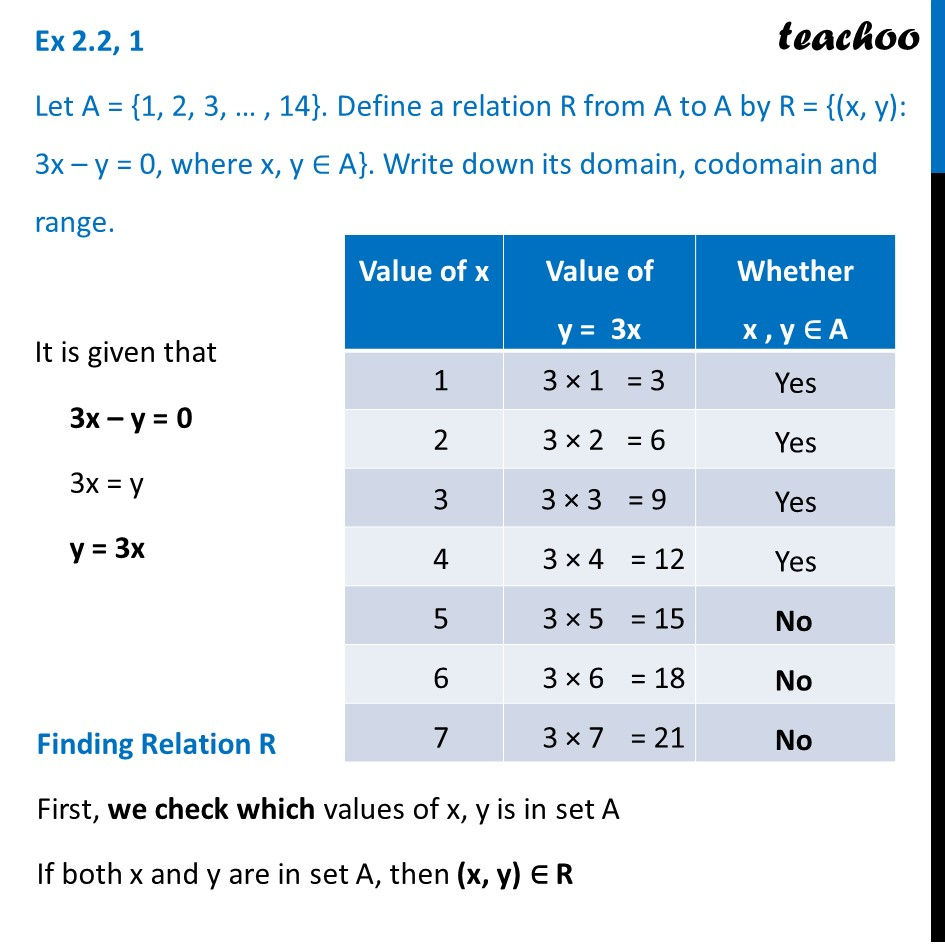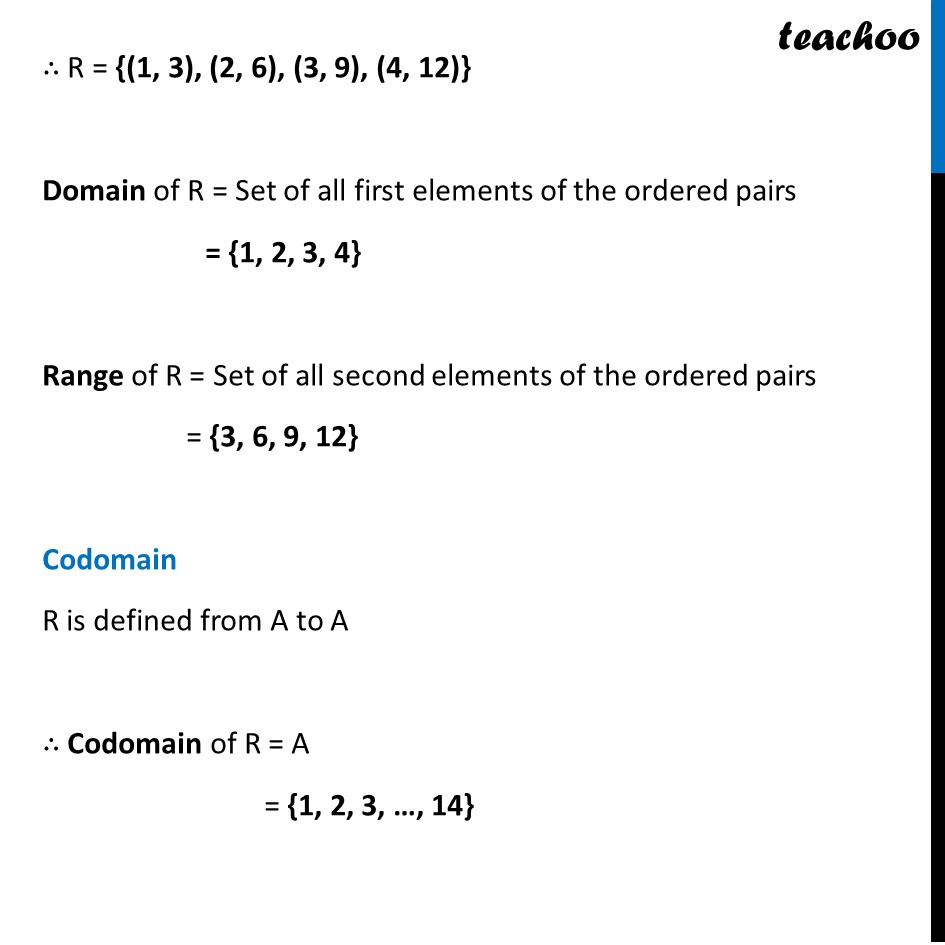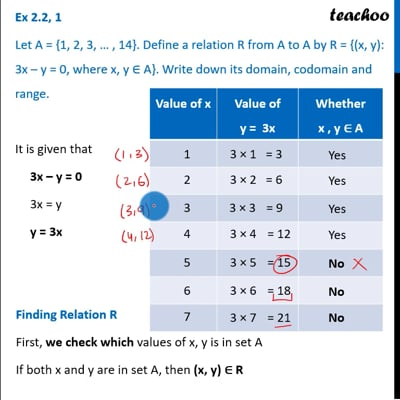Chapter 2 Class 11 Relations and Functions

Class 11
Important Questions for exams Class 11This video is only available for Teachoo black users

Solve all your doubts with Teachoo Black (new monthly pack available now!)

### Transcript

Ex 2.2, 1 Let A = {1, 2, 3, … , 14}. Define a relation R from A to A by R = {(x, y): 3x – y = 0, where x, y ∈ A}. Write down its domain, codomain and range. It is given that 3x – y = 0 3x = y y = 3x Finding Relation R First, we check which values of x, y is in set A If both x and y are in set A, then (x, y) ∈ R ∴ R = {(1, 3), (2, 6), (3, 9), (4, 12)} Domain of R = Set of all first elements of the ordered pairs = {1, 2, 3, 4} Range of R = Set of all second elements of the ordered pairs = {3, 6, 9, 12} Codomain R is defined from A to A ∴ Codomain of R = A = {1, 2, 3, …, 14}